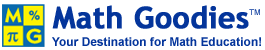# Number Theory### Introduction

Number theory is an exciting area of mathematics, with many practical applications. Some day, you may need to use factors to help you build a yard with a specific area, or use multiples to solve a scheduling problem at work. Many topics from elementary number theory are covered on Math Goodies. You have been selected to explore these topics, and to apply your knowledge through critical thinking.

You will explore resources on Math Goodies to answer specific questions on number theory. The key to learning in this WebQuest is to become engaged in finding information, and to learn from what you have found. These tasks will require a computer, access to the Internet, paper and pencil. Familiarity with Math Goodies is helpful, but not necessary.

### The Process

Process Part I: Number Theory Unit and Worksheets

1. Browse our instructional unit on Elementary Math (Number Theory), which covers factors, GCF, Multiples, LCM, prime and composite numbers, divisibility tests and exponents.
2. Complete all five exercises at the end of each core lesson in this unit.
3. Complete our Worksheet on The Sieve of Eratosthenes

Elementary Number Theory Questions

Answer each question, in order, and write down your answer on paper in your own words. You may work alone or in groups.

1. What is elementary number theory?
2. Give an example of how can multiples help you with gardening? (You may provide a specific example you found on our site.)
3. What is a factor?
4. What is 1,729,463 raised to the zero power?
5. Is 25 the same as 2 x 5? Explain why or why not using full sentences.
6. What is the Fibonacci sequence? (Hint: It can be found on several pages.)
7. Write the Fibonacci sequence on paper.
11. How did you arrive at your answer to questions 9 and question 10? What method did you use for each?
12. Why do we use divisibility tests?
13. Which test(s) will determine if the number 876 is prime or composite? Explain your answer.
14. What is the Sieve of Eratosthenes?
15. Find all prime numbers less than 100.
16. What is the smallest prime number?
17. Which prime number is even?
18. What is an emirp?
19. List all emirps between 1 and 100.

### Resources

1. Our instructional unit on Elementary Math.
2. Our worksheet on The Sieve of Eratosthenes.